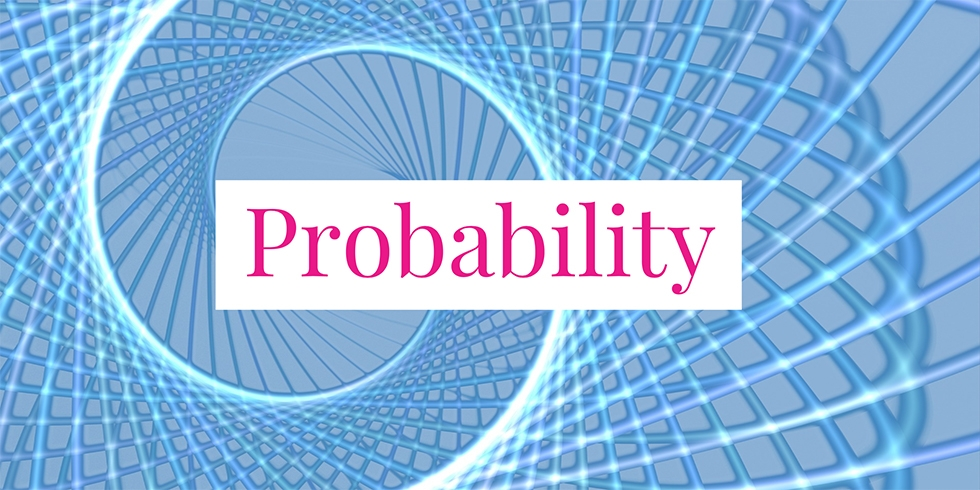# Can a Student Pass By Randomly Answering Multiple Choice Questions?## Problem 749

A final exam of the course Probability 101 consists of 10 multiple-choice questions. Each question has 4 possible answers and only one of them is a correct answer. To pass the course, 8 or more correct answers are necessary. Assume that a student has not studied probability at all and has no idea how to solve the questions. So the student decided to answer each questions randomly. Thus, for each of 10 questions, the student choose one of the 4 answers randomly and each choice is independent each other.

(1) What is the probability that the student answered correctly only one question among the 10 questions?

(2) Determine the probability that the student passes the course.

(3) What is the expected value of the number of questions the student answered correctly?

(4) Find the variance and standard deviation of the number of questions the student answered correctly.Add to solve later

## Solution.

### Solution of (1)

Let $X$ be the number of questions that the student answered correctly. For each question, the probability that the student select a correct answer is $1/4$. As selections are independent, the random variable $X$ is binomial with parameters $n=10$ and $p=1/4$. Let $P(i)$ denote the probability that the student answered $i$ questions correctly.
Then the probability that the student selects only one correct answer is given by
$P(0) = {10 \choose 1} \left(\frac{1}{4}\right)^1 \left(\frac{3}{4}\right)^9 \approx 0.1877$

### Solution of (2)

To pass the course, 8 or more correct answers are must. Thus,
\begin{align*}
&P(\text{6 or more correct answers}) = P(8) + P(9) + P(10)\6pt] &= {10 \choose 8} \left(\frac{1}{4}\right)^8 \left(\frac{3}{4}\right)^2 + {10 \choose 9} \left(\frac{1}{4}\right)^9 \left(\frac{3}{4}\right)^1 + {10 \choose 10} \left(\frac{1}{4}\right)^{10} \left(\frac{3}{4}\right)^0 \\ &\approx 0.0003862380 + 0.0000286102 + 0.0000009536 \\ &= 0.0004158018 = 0.04158018 \%. \end{align*} Hence, the desired probability is 0.0004158018 or 0.04158018%. ### Solution of (3) Recall that the expected value of a binomial random variable X with parameters (n, p) is given by \[E[X] = np. As $n=10$ and $p=1/4$, the expected value of the number of questions the student answered correctly is
$E[X] = 10 \cdot \frac{1}{4} = 2.5.$

### Solution of (4)

Recall that the variance and the standard deviation of a binomial random variable $X$ with parameters $(n, p)$ are given by
$V(X) = np(1-p) \text{ and } \sigma(X) = \sqrt{np(1-p)}.$ Substituting $n=10$ and $p=1/4$, we obtain the variance
\begin{align}
V(X) &= 10 \cdot \frac{1}{4} \cdot \frac{3}{4} = \frac{15}{8} = 1.875
\end{align}
and the standard deviation
\begin{align*}
\sigma(X) &= \sqrt{1.875} \approx 1.3693.
\end{align*}Add to solve later

### More from my site

#### You may also like...

This site uses Akismet to reduce spam. Learn how your comment data is processed.

###### More in Probability##### Given the Variance of a Bernoulli Random Variable, Find Its Expectation

Suppose that $X$ is a random variable with Bernoulli distribution $B_p$ with probability parameter $p$. Assume that the variance \$V(X)...

Close